# 互動式儀表板！Python輕鬆完成！⛵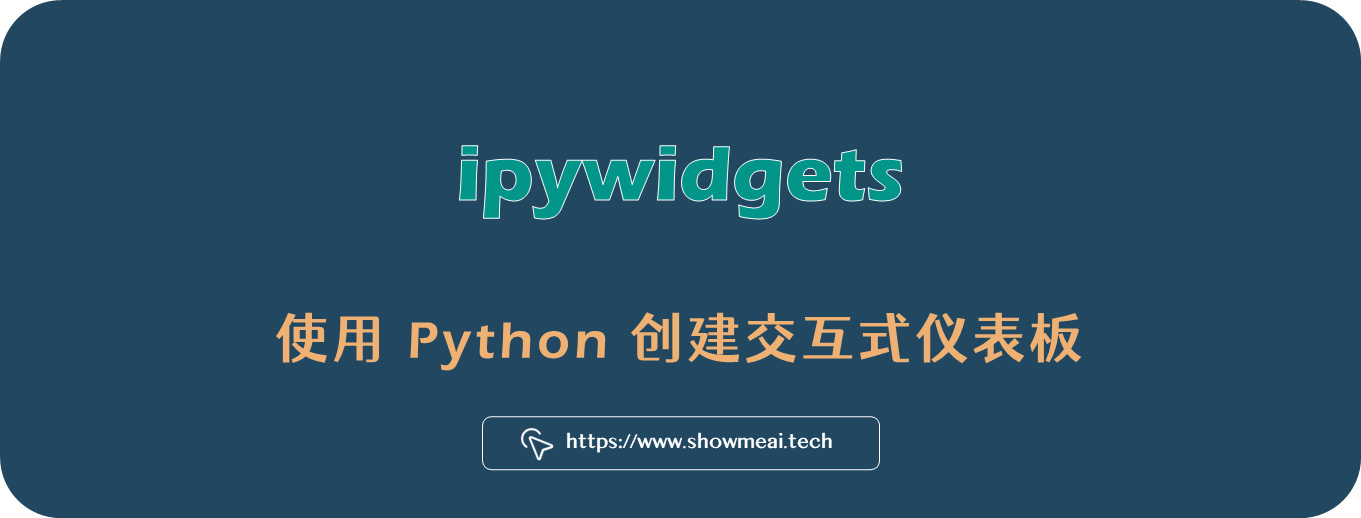# 💡 引言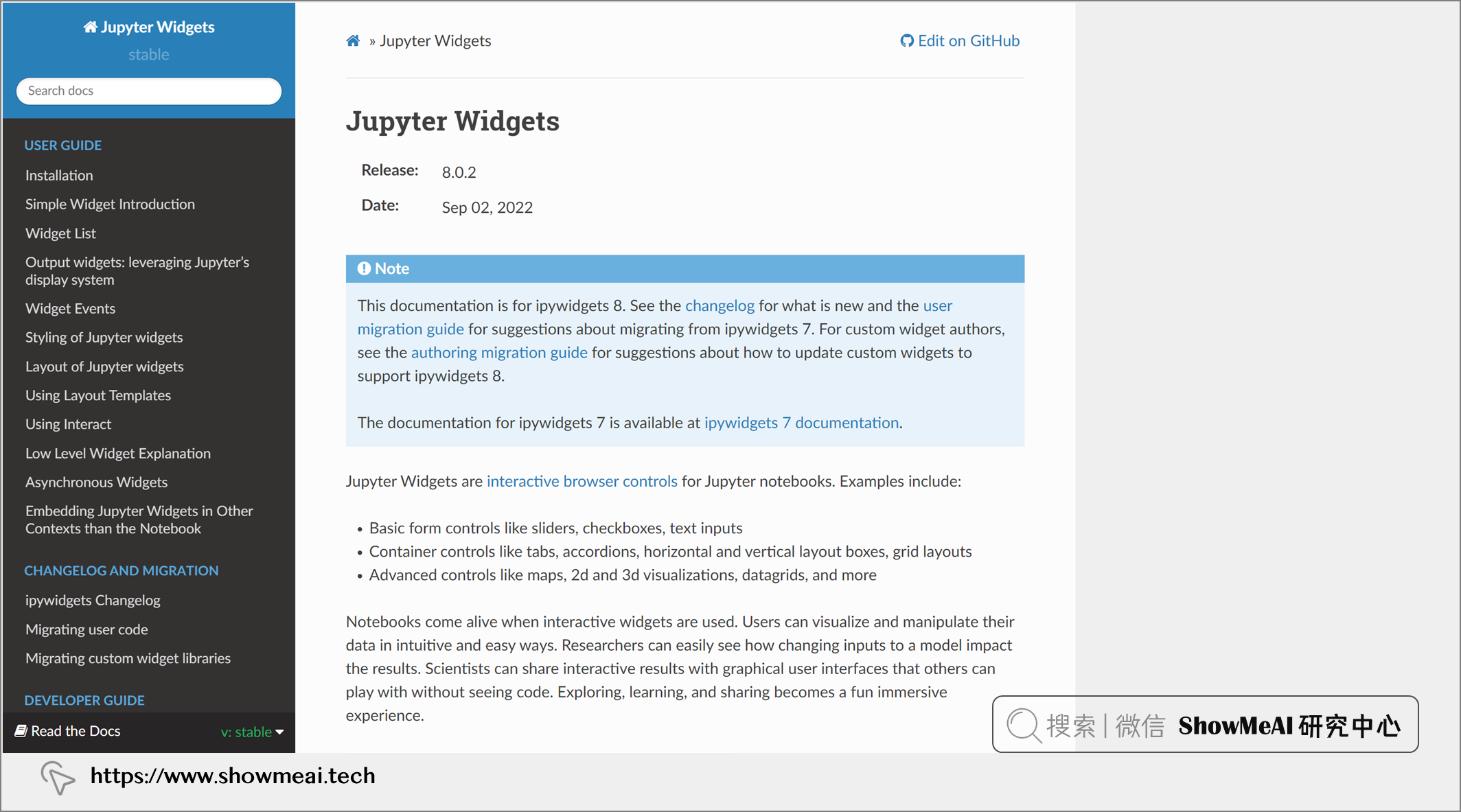🏆 實戰資料集下載（百度網盤）：公眾號『ShowMeAI研究中心』回覆『實戰』，或者點選 這裡 獲取本文 ipywidgets：使用Python建立互動式儀表板CardioGoodFitness 資料集

ShowMeAI官方GitHubhttps://github.com/ShowMeAI-Hub

```python import pandas as pd import ipywidgets import seaborn as sns import matplotlib.pyplot as plt```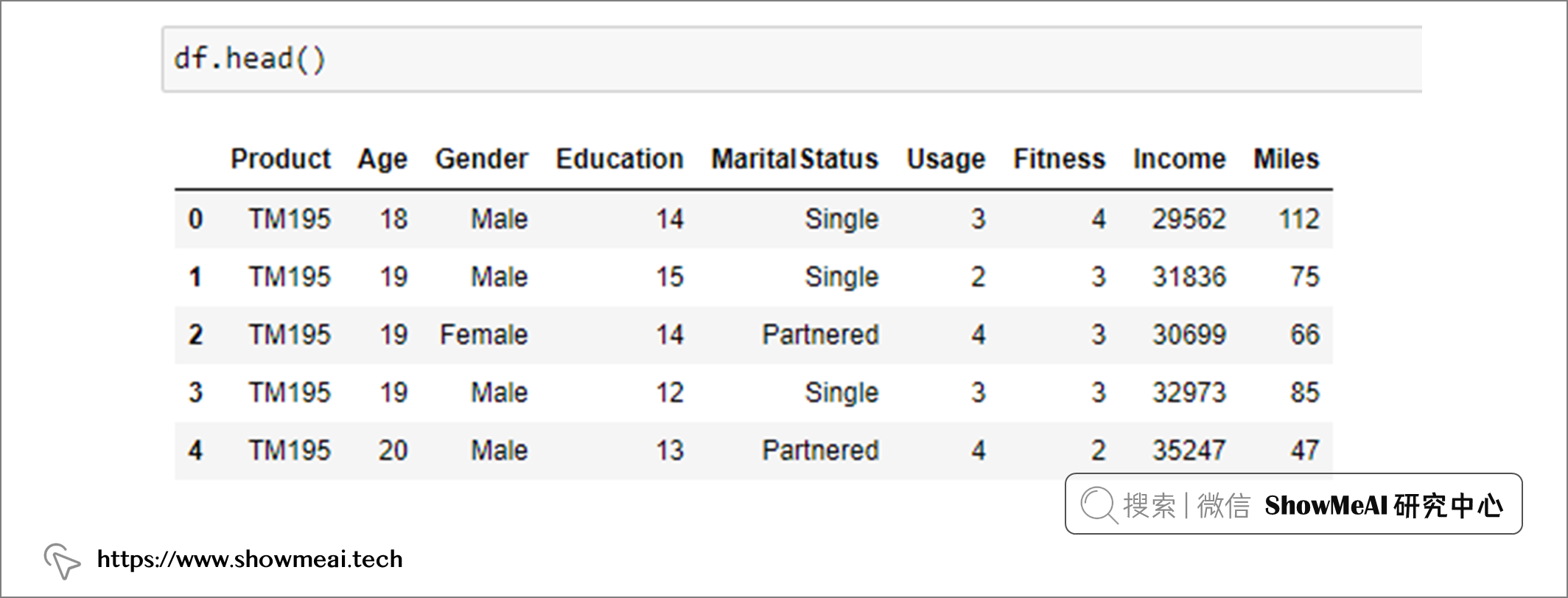# 💡 看板Demo實現：瞭解Miles的分佈

## 💦 準備工作

📘ipywidget 模組包含了很多可用的小部件。在這個演示中，我們將使用下拉框選擇類別資料，以便更好地瞭解里程分佈。我們將選擇箱線圖來繪製每個類別的里程資料。

```python %matplotlib widget

# Drop down for boxplot variable to be select

drop_down_name = ipywidgets.Dropdown(options=list(df.drop(['Miles','Income'],axis=1).columns), value=list(df.drop(['Miles','Income'],axis=1).columns), description='Columns:', disabled=False) ```

```python

# selected_vals = column used to plot

def boxplot(selected_vals): plt.close('all') fig = plt.figure(figsize=(9,5)) plt.style.use('seaborn') sns.boxplot(df[selected_vals],df['Miles']) plt.title('Boxplot of miles for' + selected_vals) plt.show() ```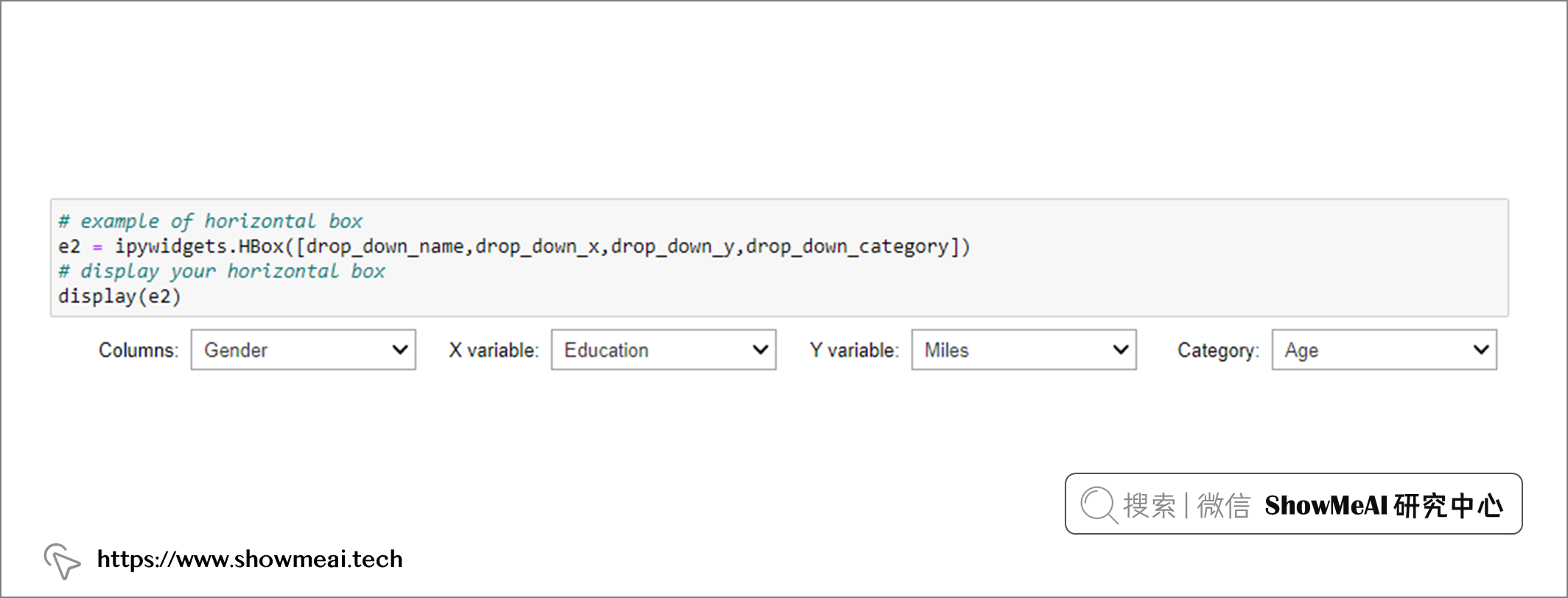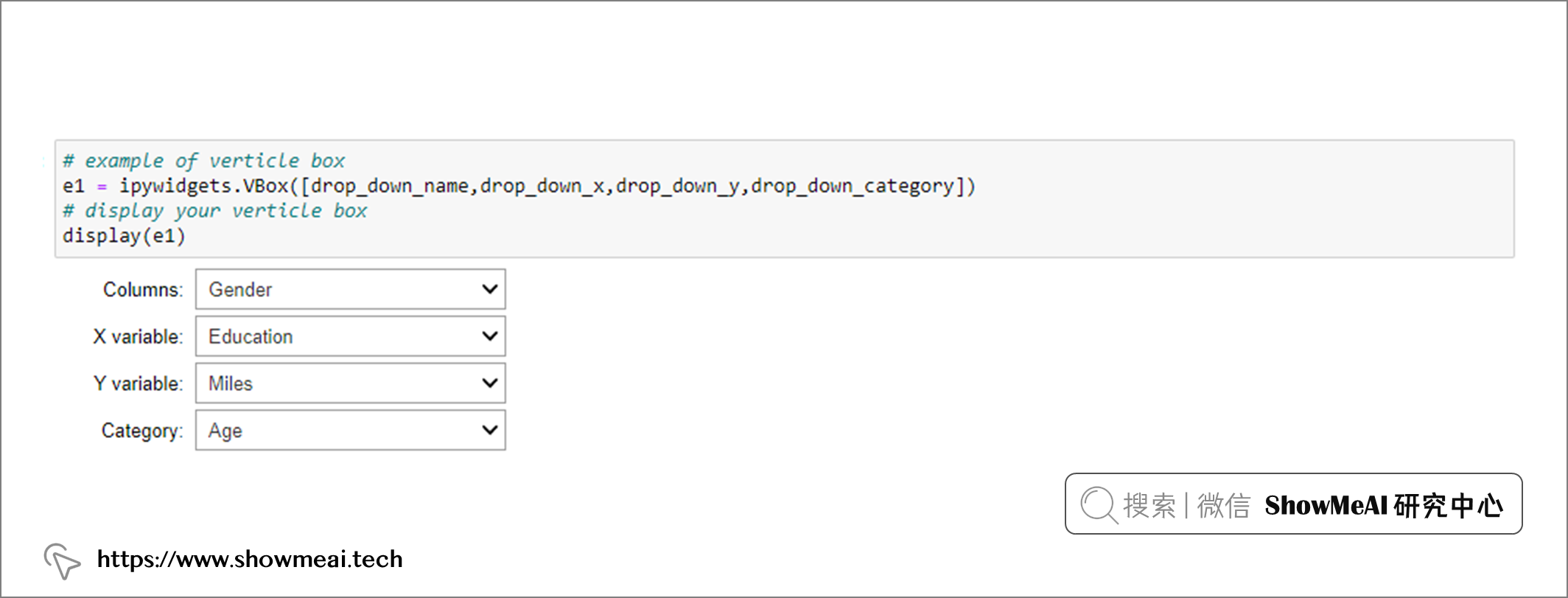```python

# layout for filtering

ui2 = ipywidgets.HBox([drop_down_name])

out2 = ipywidgets.interactive_output(boxplot, {'selected_vals' : drop_down_name})

display(ui2,out2) ```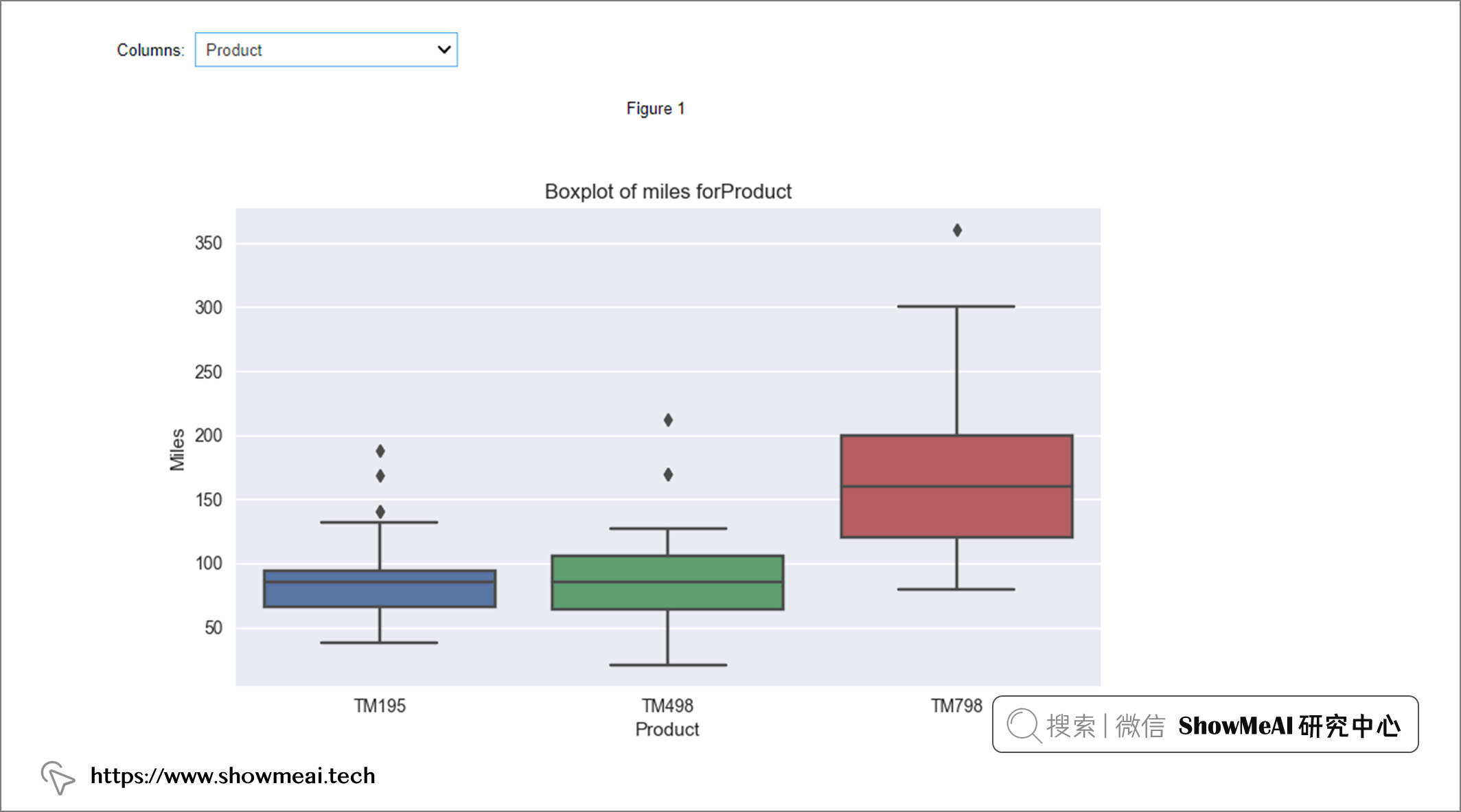```python

# dropbox select x axis

drop_down_x = ipywidgets.Dropdown(options=list(df.columns), value=list(df.columns), description='X variable:', disabled=False)

# dropbox select y axis

drop_down_y = ipywidgets.Dropdown(options=list(['Miles','Income']), value=list(['Miles','Income']), description='Y variable:', disabled=False)

# dropbox select category

drop_down_category= ipywidgets.Dropdown(options=list(df.drop(['Miles','Income'],axis=1).columns), value=list(df.drop(['Miles','Income'],axis=1).columns), description='Category:', disabled=False) ```

```python

# scatter plot function

def scatter(x,y,category): plt.close('all') fig = plt.figure(figsize=(9,5)) plt.style.use('seaborn') sns.scatterplot(data=df,x=x,y=y,hue=category) plt.title('Scatterplot of ' +x+' versus '+ y) #plt.xlabel('Date') plt.show() ```

```python

# display the layout of filtering

ui3 = ipywidgets.HBox([drop_down_x,drop_down_y,drop_down_category]) ```

```python

# related the plot link to filtering

out3 = ipywidgets.interactive_output(scatter, {'x' : drop_down_x, 'y': drop_down_y, 'category':drop_down_category}) ```

```python

# display the input and output

display(ui3,out3) ```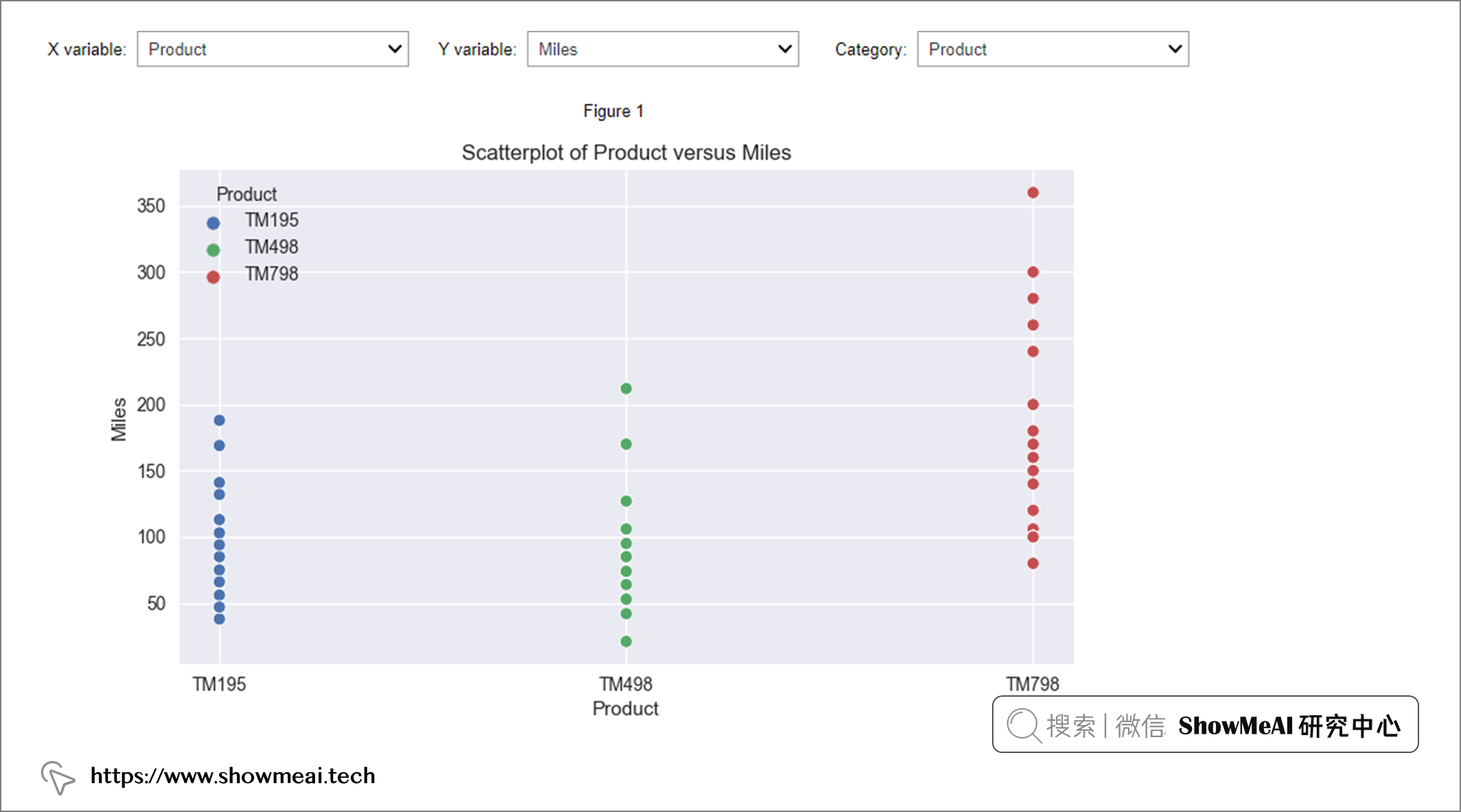# 推薦閱讀